Next: The accuracy of causal Up: Z-plane, causality, and feedback Previous: Smoothing with a triangle

# CAUSAL INTEGRATION FILTER

Begin with a function in discretized time xt. The Fourier transform with the substitution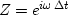is the Z-transform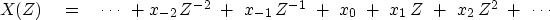(12)
Define(which will turn out to be an approximation to) by(13)
Define another signal yt with Z-transform Y(Z) by applying the operator to X(Z):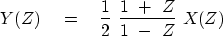(14)
Multiply both sides by (1-Z):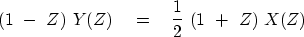(15)
Equate the coefficient of Zt on each side: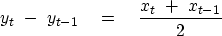(16)
Taking xt to be an impulse function, we see that yt turns out to be a step function, that is,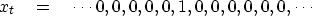(17)(18)
So yt is the discrete-domain representation of the integral of xt from minus infinity to time t. The operator (1+Z)/(1-Z) is called the bilinear transform."Next: The accuracy of causal Up: Z-plane, causality, and feedback Previous: Smoothing with a triangle
Stanford Exploration Project
10/21/1998